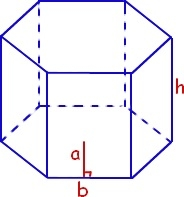# Surface Area and Volume of Hexagonal Prism in C programming

The surface area of any figure is the total area that it's surface cover.

A hexagonal prism is a three-dimensional figure that has a hexagon at both its ends. exam on prism looks like -

In mathematics, Hexagonal prism is defined as three dimensional figure with 8 faces, 18 edges, 12 vertices.Surface Area = 3ah + 3√3*(a2)
Volume = (3√3/2)a2h

## Example

#include <stdio.h>
#include<math.h>
int main() {
float a = 5, h = 10;
//Logic to find the area of hexagonal prism
float Area;
Area = 6 * a * h + 3 * sqrt(3) * a * a;
printf("Surface Area: %f",Area);
//Logic to find the Volume of hexagonal prism
float Volume;
Volume = 3 * sqrt(3) * a * a * h / 2;
printf("Volume: %f",Volume);
return 0;
}

## Output

Surface Area: 429.903809
Volume: 649.519043